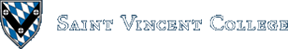Computing & Information Systems    DepartmentSearch CIS Site      Tutorials

## Software Design Using C++

### Sorting Revisited

One of the typical tasks in a Data Structures course is to compare the running times of various sort algorithms. This can be done using analysis of algorithms techniques. For example, we already know that bubblesort is Theta(n^2), but quicksort is Theta(n * lg(n)), both in the average case.

Another way to compare sort routines is to actually run several of them on the same set of test data. Rather than have to write all of the code for this ourselves, an easy way to do this is to visit Jason Harrison's sorting animation Web site. Once at this site, click on the picture for each sort routine that you wish to run. This will download a Java routine that will perform the sort and display the sorting progress visually. You will, of course, need to use a browser that is Java-enabled. Which sort performs best? Do you see an obvious speed difference between some of the sorts?

### Definitions

Recall that in a binary tree each node can have a left child node and/or a right child node. A leaf is a node with no children.

An almost complete binary tree is a binary tree in which the following 3 conditions hold: all the leaves are at the bottom level or the bottom 2 levels, all the leaves are in the leftmost possible positions, and (except possibly for the bottom level) all levels are completely filled with nodes.

Here are some examples of almost complete binary trees. Note that there is no particular ordering of the letters.

```                 R

/       \

G            A

/   \        /

T     W     G

K

/       \

L           C

/    \       /    \

B       T     Q       Z

/   \

D     P
```

The following example is a complete binary tree. (Of course, it is also fits the definition of almost complete. Any complete binary tree is automatically almost complete.)

```                  G

/     \

R         T

/   \     /   \

V     E   S     N
```

The following binary trees are not almost complete:

```                  W

/     \

T         P

/   \         \

H     K         V    (V should be pushed to the left)

M

/     \

E         L

/   \     /

I     R   Y        (this level should be completely filled)

/   \

S     C

H

/     \

N         D

/   \

R     V     (R and V should be pushed left, under N)

A

/     \

G        J     (there are leaves at 3 levels)

/   \

Y     O

/   \

B     Z
```

### What Is a Heap?

Definition: A minimal heap (descending heap) is an almost complete binary tree in which the value at each parent node is less than or equal to the values in its child nodes.

Obviously, the minimum value is in the root node. Note, too, that any path from a leaf to the root passes through the data in descending order.

Here is an example of a minimal heap:

```                  C

/     \

H         K

/   \     /

L     I   M
```

### Storage of Heap Data

The typical storage method for a heap, or any almost complete binary tree, works as follows. Begin by numbering the nodes level by level from the top down, left to right. For example, consider the following heap. The numbering has been added below the nodes.

```                  C
0
/     \

H         K
1         2
/   \     /

L     I   M
3     4   5
```

Then store the data in an array as shown below: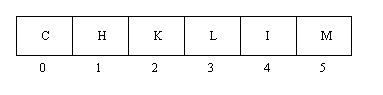The advantage of this method over using the usual pointers and nodes is that there is no wasting of space due to storing two pointer fields in each node. Instead, starting with the current index, `CI`, one calculates the index to use as follows:

 `````` Parent(CI) = (CI - 1) / 2 RightChild(CI) = 2 * (CI + 1) LeftChild(CI) = 2 * CI + 1 ``````

For example, if we start at node H (with index 1), the right child is at index 2 * (1 + 1) = 4, that is, node I.

### Inserting into a Heap

This is done by temporarily placing the new item at the end of the heap (array) and then calling a `FilterUp` routine to make any needed adjustments on the path from this leaf to the root. For example, let's insert E into the following heap:

```                  C

/     \

H         K

/   \     /

L     I   M
```

First, temporarily place E in the next available position:

```                  C

/     \

H         K

/   \     /   \

L     I   M     E
```

Of course, the new tree might not be a heap. The `FilterUp` routine now checks the parent, K, and sees that things would be out of order as they are. So K is moved down to where E was. Then the parent above that, C, is checked. It is in order relative to the target item E, so the C is not moved down. The hole left behind is filled with E, then, as this is the correct position for it.

```                  C

/     \

H         E

/   \     /   \

L     I   M     K
```

For practice, let's take the above heap and insert another item, D. First, place D temporarily in the next available position:

```                  C

/     \

H         E

/   \     /   \

L     I   M     K

/

D
```

Then the `FilterUp` routine checks the parent, L, and discovers that L must be moved down. Then the parent above that, H, is checked. It too must be moved down. Finally C is checked, but it is OK where it is. The hole left where the H had been is where the target D is then inserted.

```                  C

/     \

D         E

/   \     /   \

H     I   M     K

/

L
```

Things have now been adjusted so that we again have a heap!

### Removing from a Heap

We always remove the item from the root. That way we always get the smallest item. The problem is then how to adjust the binary tree so that we again have a heap (with one less item).

The algorithm works like this: First, remove the root item and replace it temporarily with the item in the last position. Call this replacement the target. A `FilterDown` routine is then used to check the path from the root to a leaf for the correct position for this target. The path is chosen by always selecting the smaller child at each node. For example, let's remove the C from this heap:

```                  C

/     \

D         E

/   \     /   \

H     I   M     K

/

L
```

First we remove the C and replace it with the last item (the target), L:

```                  L

/     \

D         E

/   \     /   \

H     I   M     K
```

The smaller child of L is D. Since D is out of order compared to the target L, we move D up. The smaller child under where D had been is H. When H is compared to L we see that the H too needs to be moved up. Since we are now at a leaf, this empty leaf is where the target L is put.

```                  D

/     \

H         E

/   \     /   \

L     I   M     K
```

For another example, let's remove the E from the following heap:

```                  E

/     \

G         K

/   \     /   \

J      N   K     X

/ \    /

X   Y  P
```

First remove the E and replace it with the target P (the last item):

```                  P

/     \

G         K

/   \     /   \

J      N   K     X

/ \

X   Y
```

Now use the `FilterDown` routine to filter the P down to its correct position by checking the smaller child, G, which should be moved up, and then the smaller child below that, J, which should also be moved up. Finally, the smaller child, X, under where J had been is checked, but it does not get moved since it is OK relative to the target P. The P is then placed in the empty node above X. We then have the following heap:

```                  G

/     \

J         K

/   \     /   \

P      N   K     X

/ \

X   Y
```

### Comic Relief

Thanks to http://xkcd.com. Note their license information.### Heapsort

Heapsort is performed by somehow creating a heap and then removing the data items one at a time. The heap could start as an empty heap, with items inserted one by one. However, there is a relatively easy routine to convert an array of items into a heap, so that method is often used. This routine is described below. Once the array is converted into a heap, we remove the root item (the smallest), readjust the remaining items into a heap, and place the removed item at the end of the heap (array). Then we remove the new item in the root (the second smallest), readjust the heap, and place the removed item in the next to the last position, etc.

Heapsort is Theta(n * lg(n)), either average case or worst case. This is great for a sorting algorithm! No appreciable extra storage space is needed either. On average, quicksort (which is also Theta(n * lg(n)) for the average case) is faster than heapsort. However, quicksort has that bad Theta(n^2) worst case running time.

#### Example Trace of Heapsort

Let's heapsort the following array: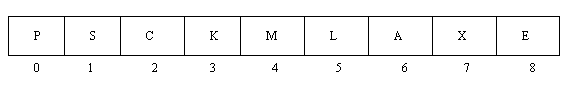To convert this to a heap, first go to the index of the last parent node. This is given by (HeapSize - 2) / 2. In this case, (9 - 2) / 2 = 3. Thus K is the last parent in the tree. We then apply the `FilterDown` routine to each node from this index down to index 0. (Note that this is each node from 3 down to 0, not just the nodes along the path from index 3 to index 0.)

In our example, the array corresponds directly to the following binary tree. Note that this is not yet a heap.

```                  P

/     \

S         C

/   \     /   \

K      M   L     A

/ \

X   E
```

Applying `FilterDown` at K gives the following. (Note that E is the smaller child under K.)

```                  P

/     \

S         C

/   \     /   \

E      M   L     A

/ \

X   K
```

Now apply `FilterDown` at index 2, that is, at node C. (Under C, A is the smaller child.)

```                  P

/     \

S         A

/   \     /   \

E      M   L     C

/ \

X   K
```

Next, apply `FilterDown` at index 1, that is, at node S. Check the smaller child, E, and then the smaller child under that, namely K. Both E and K get moved up.

```                  P

/     \

E         A

/   \     /   \

K      M   L     C

/ \

X   S
```

Finally, apply `FilterDown` at index 0, that is, at the root node. We check the smaller child, A, and then the smaller child, C, relative to the target P. Both A and C get moved up.

```                  A

/     \

E         C

/   \     /   \

K      M   L     P

/ \

X   S
```

Now we have a heap! The first main step of heapsort has been completed. The other main component of heapsort was described earlier: to repeatedly remove the root item, adjust the heap, and put the removed item in the empty slot toward the end of the array (heap).

First we remove the A, adjust the heap by using `FilterDown` at the root node, and place the A at the end of the heap (where it is not really part of the heap at all and so is not drawn below as connected to the tree).

```                  C         (the target is S)

/     \

E         L

/   \     /   \

K      M   S     P

/ .

X   A
```

Of course, all of this is really taking place in the array that holds the heap. At this point it looks like the following. Note that the heap is stored from index 0 to index 7. The A is after the end of the heap.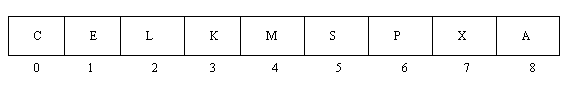Next we remove the C, adjust the heap by using `FilterDown` at the root node, and place the C at the end of the heap:

```                  E         (the target is X)

/     \

K         L

/   \     /   \

X      M   S     P

. .

C   A
```

Next we remove the E, adjust the heap by using `FilterDown` at the root node, and place the E at the end of the heap:

```                  K         (the target is P)

/     \

M         L

/   \     /   .

X      P   S     E

. .

C   A
```

Next we remove the K, adjust the heap by using `FilterDown` at the root node, and place the K at the end of the heap:

```                  L         (the target is S)

/     \

M         S

/   \     .   .

X      P   K     E

. .

C   A
```

Next we remove the L, adjust the heap by using `FilterDown` at the root node, and place the L at the end of the heap:

```                  M         (the target is P)

/     \

P         S

/   .     .   .

X      L   K     E

. .

C   A
```

Next we remove the M, adjust the heap by using `FilterDown` at the root node, and place the M at the end of the heap:

```                  P         (the target is X)

/     \

X         S

.   .     .   .

M      L   K     E

. .

C   A
```

Next we remove the P, adjust the heap by using `FilterDown` at the root node, and place the P at the end of the heap:

```                  S         (the target is S)

/     .

X         P

.   .     .   .

M      L   K     E

. .

C   A
```

Next we remove the S, adjust the heap (now a trivial operation), and place the S at the end of the heap:

```                  X         (the target is X)

.     .

S         P

.   .     .   .

M      L   K     E

. .

C   A
```

Since only the item X remains in the heap, and since we have removed the smallest item, then the second smallest, etc., the X must be the largest item and should be left where it is. If you now look at the array that holds the above items you will see that we have sorted the array in descending order: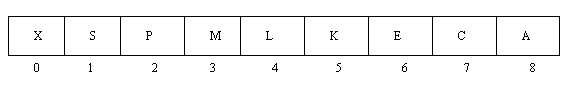#### Complete Code for Heapsort

You can easily see in this example that a `HeapClass` class has been set up with a constructor and functions for inserting and removing an item. An object of this class contains three data fields: the maximum heap size, the actual heap size (number of items currently in the heap), and a pointer to an array containing the heap data. Note that the array itself is located outside of the object. This may not be the cleanest approach, but it is useful since we are going to start with an ordinary array and sort it via heapsort. Why have a second array inside of the object, when this array would hold the very same data?

The `FilterDown` and `FilterUp` routines are private functions of this class. Three short private functions are also used to find the index of the parent node, the index of the left child, and the index of the right child. When you place the code for a function inside the class definition as has been done here, you automatically get an inline function. Thus these functions will execute very efficiently, without the usual function call and return overhead.

In the code for the `Delete` function (and elsewhere), note that we use the fact that we can use array subscripting on a pointer to the first item in an array, since an array name is just a pointer to the first item. Thus you see lines such as the following, which use the pointer field that is part of the `HeapClass` object.

 `````` Temp = HeapArrayPtr; ``````

Once `HeapClass` has been set up, it is very easy to write the code for heapsort. In our example it has been written as a stand-alone function as shown below:

 `````` void HeapSort(IntArrayType IntArray, int Count) { int Smallest, k; HeapClass H(IntArray, Count); for (k = Count - 1; k >= 1; k--) { Smallest = H.Delete(); IntArray[k] = Smallest; } } ``````

Remember that even after the object H goes out of existence at the end of the above function, the heap data is still sitting in `IntArray` and is now in descending order. Thus, sorting of the array has been accomplished.

### References:

 Data Structures Using Pascal, 2nd ed. Aaron M. Tenenbaum, Moshe J. Augenstein. Prentice-Hall (1986). See pages 396 through 405. Data Structures with C++. William Ford, William Topp. Prentice-Hall (1996). See pages 690 through 703. Introduction to Algorithms. Thomas H. Cormen, Charles E. Leiserson, and Ronald L. Rivest. McGraw-Hill (1990). Chapter 7 covers heaps and heapsort.

### Related Item

Binary Trees

Back to the main page for Software Design Using C++

Author: Br. David Carlson with contributions by Br. Isidore Minerd
Last updated: April 20, 2011
Disclaimer